### Home > A2C > Chapter 10 > Lesson 10.1.1 > Problem10-19

10-19.
1. One way to win in a game with two dice is to roll a sum of six before getting a sum of seven. (Anything else that happens ” sums of 2, 3, 4, 5, 8, 9, 10, 11, or 12 - are ignored.) Homework Help ✎

1. How many ways are there to get a sum of six?

2. How many ways are there to get seven?

3. How many possible outcomes are important in this problem?

4. What is the probability of getting a six before a seven?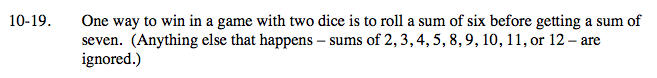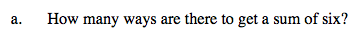5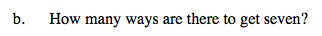6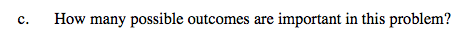Do the other sums have any effect on the probability of winning the game?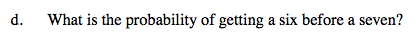What fraction of the important outcomes are sums of six?